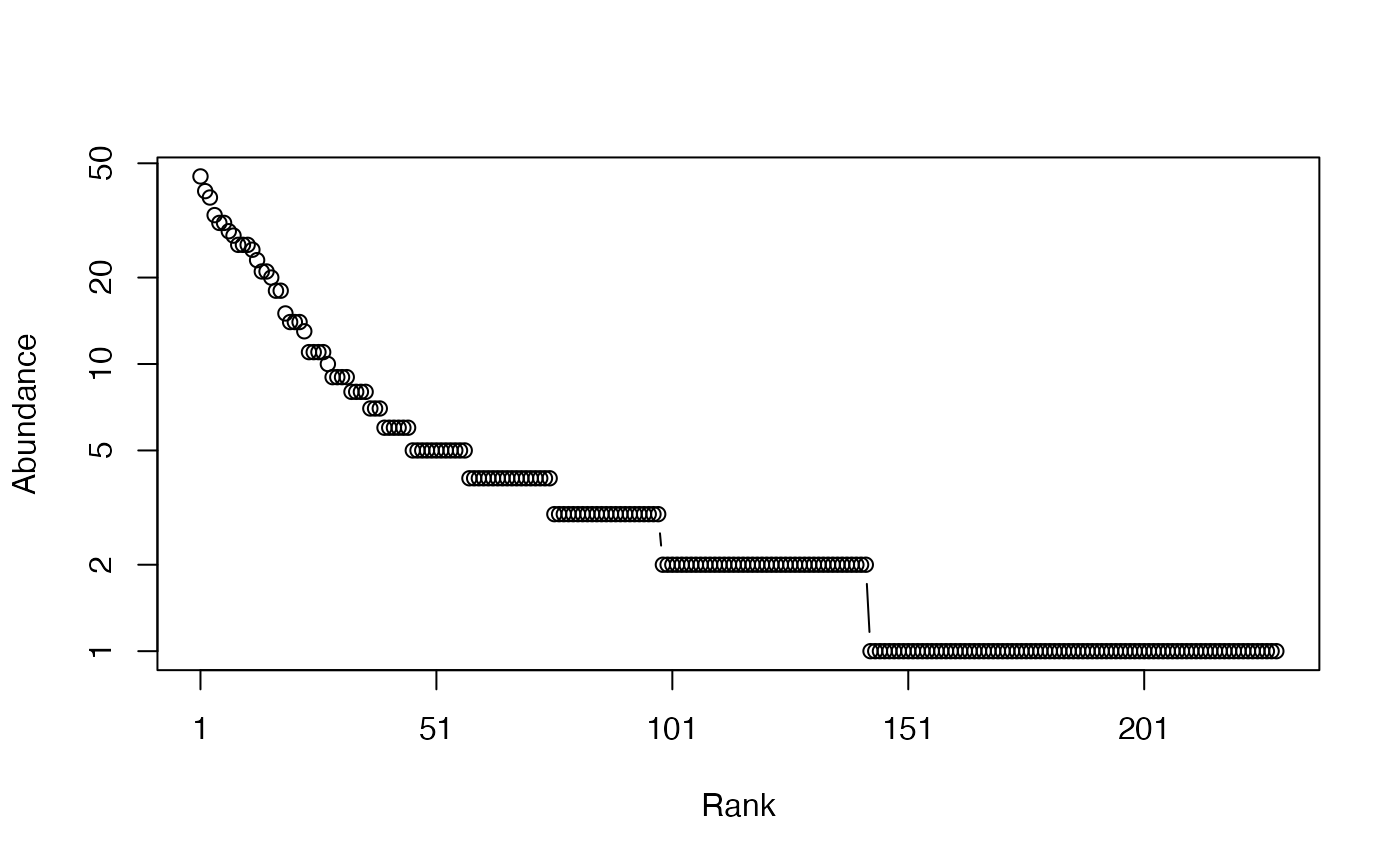Calculates the Generalized Simpson's entropy of order $$r$$ of a probability or abundance vector, and its effective number of species.

GenSimpson(NorP, r = 1, ...)
bcGenSimpson(Ns, r = 1, CheckArguments = TRUE)
# S3 method for ProbaVector
GenSimpson(NorP, r = 1, ...,
CheckArguments = TRUE, Ps = NULL)
# S3 method for AbdVector
GenSimpson(NorP, r = 1, ...,
CheckArguments = TRUE, Ns = NULL)
# S3 method for integer
GenSimpson(NorP, r = 1, ...,
CheckArguments = TRUE, Ns = NULL)
# S3 method for numeric
GenSimpson(NorP, r = 1, ...,
CheckArguments = TRUE, Ps = NULL, Ns = NULL)
GenSimpsonD(NorP, r = 1, ...)
bcGenSimpsonD(Ns, r = 1, CheckArguments = TRUE)
# S3 method for ProbaVector
GenSimpsonD(NorP, r = 1, ...,
CheckArguments = TRUE, Ps = NULL)
# S3 method for AbdVector
GenSimpsonD(NorP, r = 1, ...,
CheckArguments = TRUE, Ns = NULL)
# S3 method for integer
GenSimpsonD(NorP, r = 1, ...,
CheckArguments = TRUE, Ns = NULL)
# S3 method for numeric
GenSimpsonD(NorP, r = 1, ...,
CheckArguments = TRUE, Ps = NULL, Ns = NULL)

## Arguments

Ps

A probability vector, summing to 1.

Ns

A numeric vector containing species abundances.

NorP

A numeric vector, an integer vector, an abundance vector (AbdVector) or a probability vector (ProbaVector). Contains either abundances or probabilities.

r

A number: the order of diversity. Default is 1 for Simpson's diversity.

...

CheckArguments

Logical; if TRUE, the function arguments are verified. Should be set to FALSE to save time when the arguments have been checked elsewhere.

## Details

The Generalized Simpson's Entropy (Zhang and Zhou, 2010) of order $$r$$ is, in the species accumulation curve, the probability for the individual sampled in rank $$r+1$$ to belong to a new species. It is a measure of diversity so long as $$r$$ is lower than the number of species (Grabchak et al., 2016).

Bias correction requires the number of individuals. Use bcGenSimpson. It is limited to orders $$r$$ less than or equal to the number of individuals in the community.

The effective number of species GenSimpsonD (explicit diversity) has been derived by Grabchak et al. (2016).

The functions are designed to be used as simply as possible. GenSimpson is a generic method. If its first argument is an abundance vector, an integer vector or a numeric vector which does not sum to 1, the bias corrected function bcGenSimpson is called. Explicit calls to bcGenSimpson (with bias correction) or to GenSimpson.ProbaVector (without correction) are possible to avoid ambiguity. The .integer and .numeric methods accept Ps or Ns arguments instead of NorP for backward compatibility.

## Value

A named number equal to the calculated index or diversity. The name is either "Biased" or "Unbiased", depending on the estimator used.

## Note

The unbiased estimator is calculated by the GenSimp.z function of the EntropyEstimation package.

## Examples

# Load Paracou data (number of trees per species in two 1-ha plot of a tropical forest)
data(Paracou618)
# Ns is the total number of trees per species
Ns <- as.AbdVector(Paracou618.MC$Ns) # Species probabilities Ps <- as.ProbaVector(Paracou618.MC$Ns)
# Whittaker plot
plot(Ns)# Calculate GenSimpson entropy of order 1, equal to Simpson's index of diversity
GenSimpson(Ps, 1)
#>    Biased
#> 0.9854485
# Calculate an unbiased estimator of GenSimpson diversity of order 100
GenSimpsonD(Ns, 100)
#> Unbiased
#> 115.9597